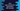# What is a copy constructor in Java - Explanation with example### What is a copy constructor in Java : explanation with example :

A copy constructor is like a normal constructor we use in Java classes. Only difference is that this constructor takes one object of the same class and then assign values to the variables of the class.

For example, suppose you have one class with 10 private variables. We can create one constructor with 10 different parameters or we can create one constructor with only one parameter , i.e. the object of the same class. The main advantage of the second method is that if we add any more variables to the class in future, we don’t have to change the constructor. Let’s try to understand it with an example :

### Example Program :

``````//1
class Student {
private String studentName;
private int studentAge;

public Student(String name, int age) {
this.studentName = name;
this.studentAge = age;
}

//2
Student(Student student) {
this.studentName = student.studentName;
this.studentAge = student.studentAge;
}

//3
public String getStudentInfo() {
return "Name : " + studentName + ",Age : " + studentAge;
}
}

public class New {
public static void main(String[] args) {
//4
Student s = new Student("Albert", 10);
Student student = new Student(s);

//5
System.out.println(student.getStudentInfo());
}
}``````

### Explanation :

The commented numbers in the above program denote the step number below :

1. Student class is our class containing the copy constructor. It has two private variables.
2. Student(Student student) constructor is the copy constructor. It takes one object of type Student and then assign its values to the private variables.
3. This function is used to print the name and age of the student.
4. Create one object of type Student and pass it to the copy constructor of Student. Then assign its values to the local variables.
5. Finally, call the getStudentInfo() function to print the name and age of the student object.